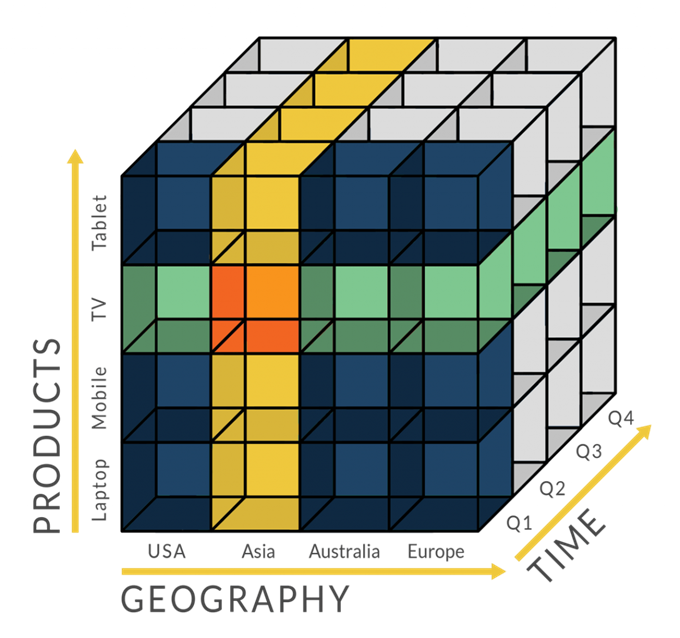# 数据可视化之直方图

## 直方图简介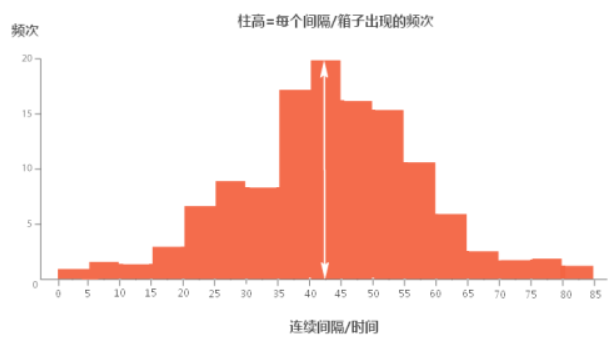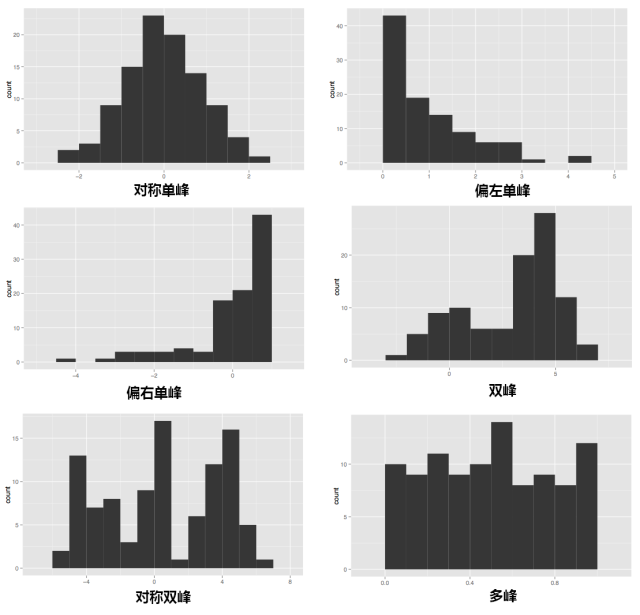### 直方图与柱状图的差别

1.直方图展示数据的分布，柱状图比较数据的大小。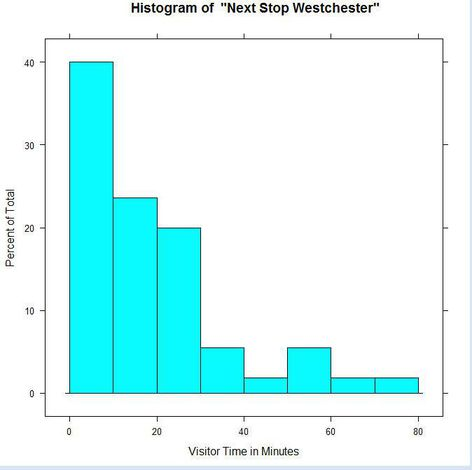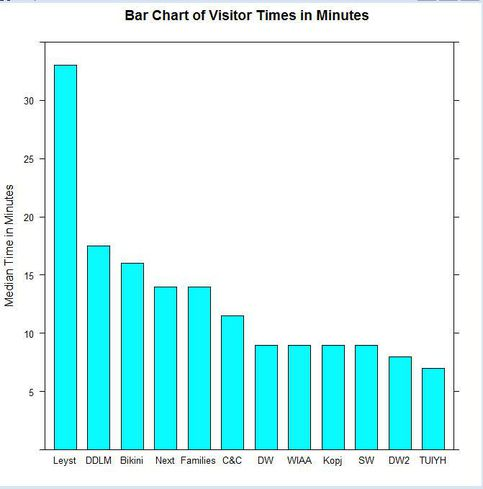2.直方图X轴为定量数据，柱状图X轴为分类数据。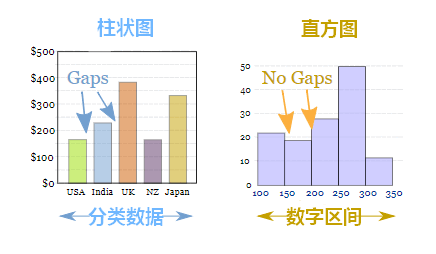3.直方图柱子无间隔，柱状图柱子有间隔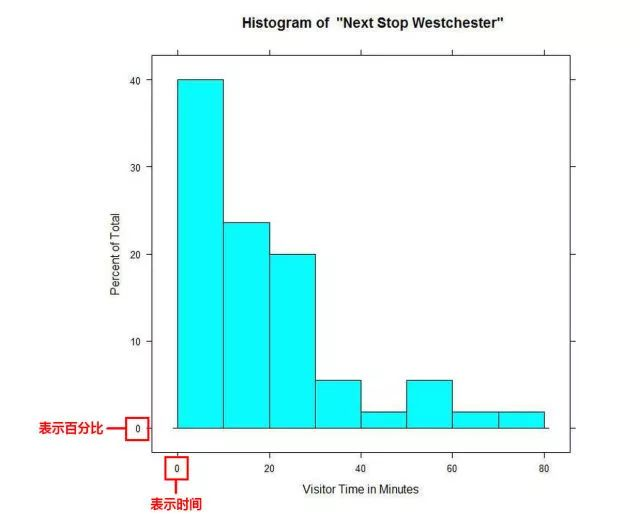4.直方图柱子宽度可不一，柱状图柱子宽度须一致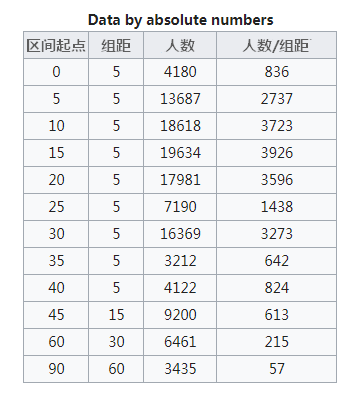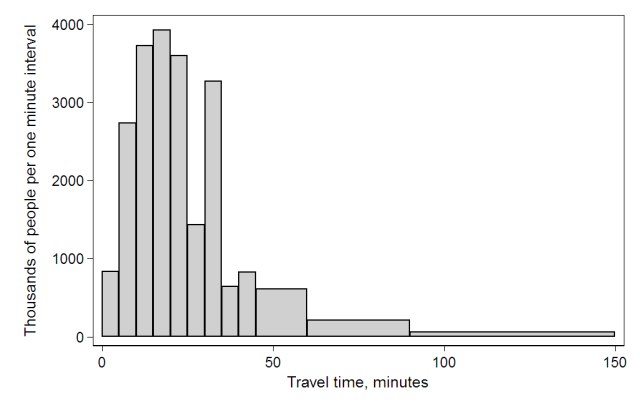## 直方图绘制

numpy.histogram(a, bins=10, range=None, normed=None, weights=None, density=None)

• a：array_like，需要处理的数据
• bins：int或序列或str
• 如果是int，则数据会进行按给定的值平均划分
• 如果给定的序列，则按序列的给定的数值进行边界划分（包括右边值）
• 如果传入的是字符串，则使用histogram_bin_edges定义的字符串类型
• range(float, float)：可选，设定需要划分的数据的范围，默认为(a.min(), a.max())
• weights：array_like，可选，给字符串的权重
• density：布尔类型，如果为False，则显示具体的数量，如果为True则显示占比

### numpy.histogram_bin_edges定义的字符串类型

• auto：sturges 或fd 估算器中较大的那个值。提供良好的全方位性能。
• sqrt：使用的平方根(数据大小)估计量，以求其速度和简便性。公式：$n_h = \sqrt{n}$
• rice：估计器不考虑可变性，仅考虑数据大小。通常会高估所需的箱数量。公式：$n_h = 2\sqrt{n}$
• sturges：R的默认方法，仅考虑数据大小。仅对高斯数据最佳，而对于大型非高斯数据集则低估了bin的数量。公式：$n_h = \log_{2}{n}+1$
• doane：Sturges估算器的改进版，可以更好地与非正常数据集配合使用。$n_h = 1+\log_{2}{n}+\log_{2}{1+\frac{\left | g_1\right |}{\sigma _{g_1}}},g_1=mean[(\frac{x-u}{\sigma })^3],\sigma _{g_1}=\sqrt{\frac{6(n-2)}{(n+1)(n+3)}}$
• fd： Freedman Diaconis Estimator，考虑到数据可变性和数据大小的稳健(对异常值具有弹性)的估计器。公式:$h = 2\frac{IQR}{n^{1/3}}$，其中IQR为四分位距，详细介绍见箱形图简介
• scott：考虑数据变异性和数据大小的鲁棒估计器。公式：$h = \sigma \sqrt{\frac{24\ast \sqrt{\pi }}{n}}$， 与标准差成正比，与数据量立方根成反比，对于小数据集来说可能过于保守，但对于大数据集来说非常好。标准差对异常值不是很稳健，在没有异常值的情况下，值与Freedman-Diaconis估计非常相似。
• stone：基于平方根误差的leave-one-out cross-validation（留一交叉验证）估计器。可以看作是Scott规则的概括。

### 留一交叉验证

$$\frac{1}{N}\sum_{n=1}^{N}e_n=\frac{1}{N}\sum_{n=1}^{N}err(g_n^-(x_n),y_n)$$

• 数据集少，如果像正常一样划分训练集和验证集进行训练，那么可以用于训练的数据本来就少，还被划分出去一部分，这样可以用来训练的数据就更少了。loocv可以充分的利用数据。
• 因为loocv需要划分N次，产生N批数据，所以在一轮训练中，要训练出N个模型，这样训练时间就大大增加。所以loocv比较适合训练集较少的场景

\begin{aligned}\mathcal{E} E_{\mathrm{loocv}}(\mathcal{H}, \mathcal{A})=\sum_{\mathcal{D}} \frac{1}{N} \sum_{n=1}^{N} e_{n} &=\frac{1}{N} \sum_{n=1}^{N} \mathcal{E}_{\mathcal{D}} e_{n} \\ &=\frac{1}{N} \sum_{n=1}^{N} \sum_{\mathcal{D}_{n}\left(\mathbf{x}_{n}, y_{n}\right)} \sum_{\mathrm{Prr}}\left(g_{n}^{-}\left(\mathbf{x}_{n}\right), y_{n}\right) \\ &=\frac{1}{N} \sum_{n=1}^{N} \sum_{\mathcal{D}_{n}} E_{\mathrm{out}}\left(g_{n}^{-}\right) \\ &=\frac{1}{N} \sum_{n=1}^{N} \bar{E}_{\mathrm{out}}(N-1)=\overline{E_{\mathrm{out}}}(N-1)\end{aligned}

• $\varepsilon_{D}$表示在不同数据集上的期望，$\varepsilon$是表示期望的符号，这里不同的数据D,我的理解是对原始数据的N次划分得到的不同数据集
• $\varepsilon_{D}e_{n}=> \varepsilon_{D_{n}}\varepsilon_{{(x_{n},y_{n})}}e_{n}$​是因为$D_{n}$和$(x_{n},y_{n})$服从iid分布
• $\varepsilon_{{(x_{n},y_{n})}}err(g_{n}^{-}(x_{n}),y_{n})$转化为$E_{out}(g_{n}^{-})$是期望的定义和$E_{out}$的定义，虽然是对剩下的一个求误差，但是求的是对剩下单个数据的期望，单个数据的的期望相当于对所有未知数据上求概率平均（因为训练数据和位置数据是iid,所以对训练数据求期望相当终于对未知数据求期望），也就是$g_{n}^{-}$在未知数据上的E即$E_{out}$

LOOCV的优点：

• 充分利用数据
• 因为采样是确定的，所以最终误差也是确定的，不需要重复LOOCV

LOOCV的缺点：

• 训练起来耗时
• 由于每次只采一个样本作为验证，导致无法分层抽样，影响验证集上的误差。举个例子，数据集中有数量相等的两个类，对于一条随机数据，他的预测结果是被预测为多数的结果，如果每次划出一条数据作为验证，则其对应的训练集中则会少一条，导致训练集中该条数据占少数从而被预测为相反的类，这样原来的误差率为50%，在LOOCV中为100%

LOOCV模型选择问题：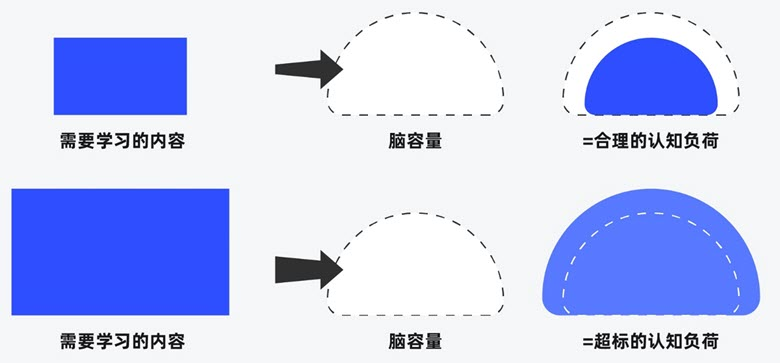##### 界面设计和游戏设计中的认知负荷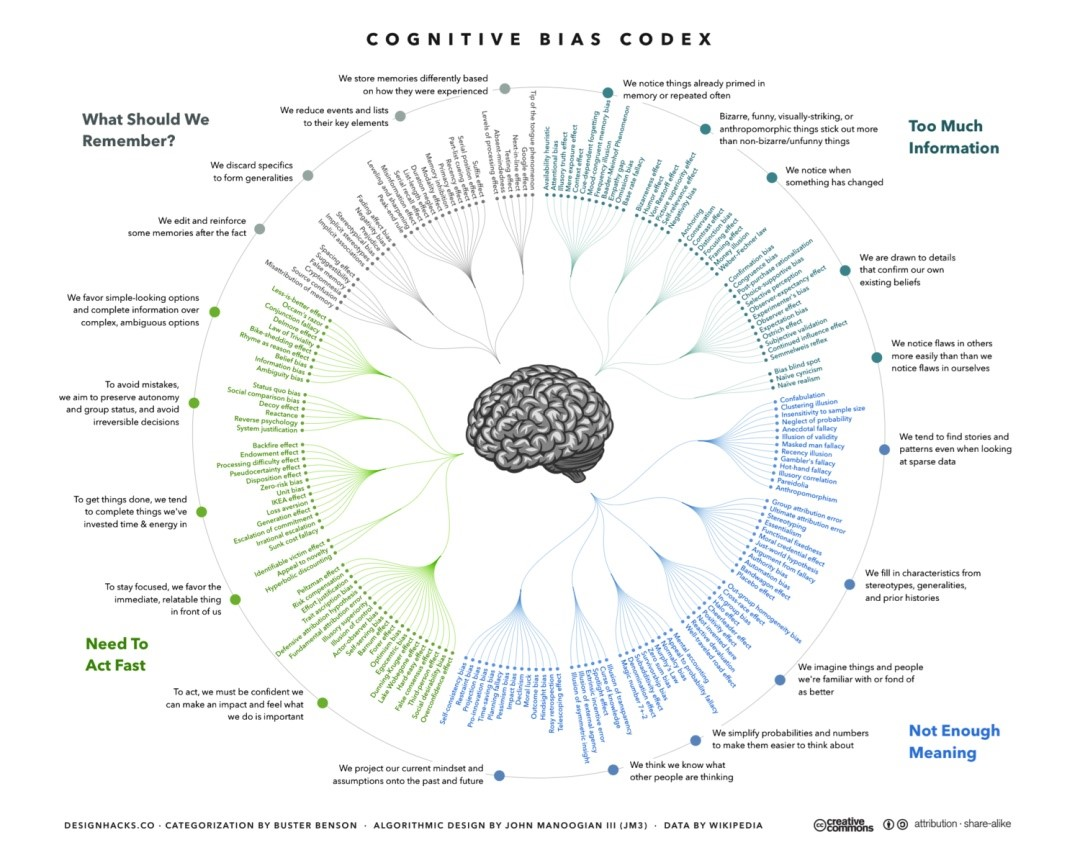##### 理解认知偏差